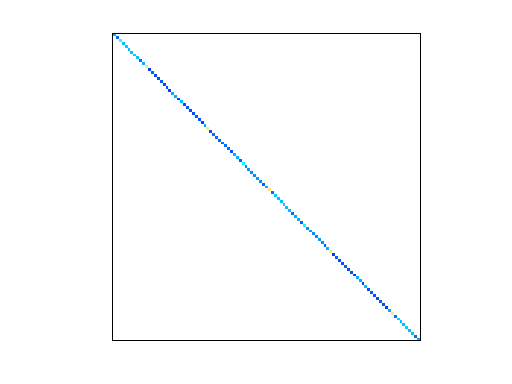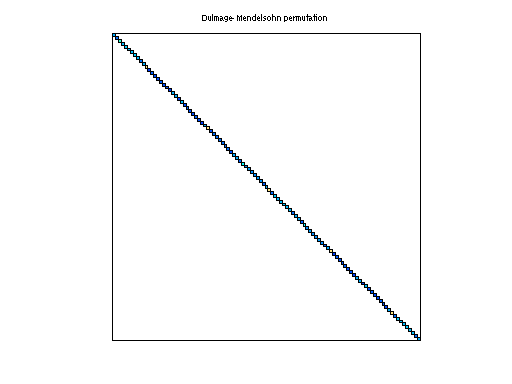Matrix: HB/bcsstm06

Description: SYMMETRIC MASS MATRIX, MEDIUM TEST PROBLEM, LUMPED MASSES(undirected graph drawing)• Matrix group: HB
• download as a MATLAB mat-file, file size: 2 KB. Use UFget(61) or UFget('HB/bcsstm06') in MATLAB.

 Matrix properties number of rows 420 number of columns 420 nonzeros 420 structural full rank? yes structural rank 420 # of blocks from dmperm 420 # strongly connected comp. 420 explicit zero entries 0 nonzero pattern symmetry symmetric numeric value symmetry symmetric type real structure symmetric Cholesky candidate? yes positive definite? yes

 author J. Lewis editor I. Duff, R. Grimes, J. Lewis date 1982 kind structural problem 2D/3D problem? yes

 Ordering statistics: result nnz(chol(P*(A+A'+s*I)*P')) with AMD 420 Cholesky flop count 4.2e+02 nnz(L+U), no partial pivoting, with AMD 420 nnz(V) for QR, upper bound nnz(L) for LU, with COLAMD 420 nnz(R) for QR, upper bound nnz(U) for LU, with COLAMD 420

 SVD-based statistics: norm(A) 7602.41 min(svd(A)) 0.00219908 cond(A) 3.45708e+06 rank(A) 420 sprank(A)-rank(A) 0 null space dimension 0 full numerical rank? yes

 singular values (MAT file): click here SVD method used: s = svd (full (A)) ; status: ok